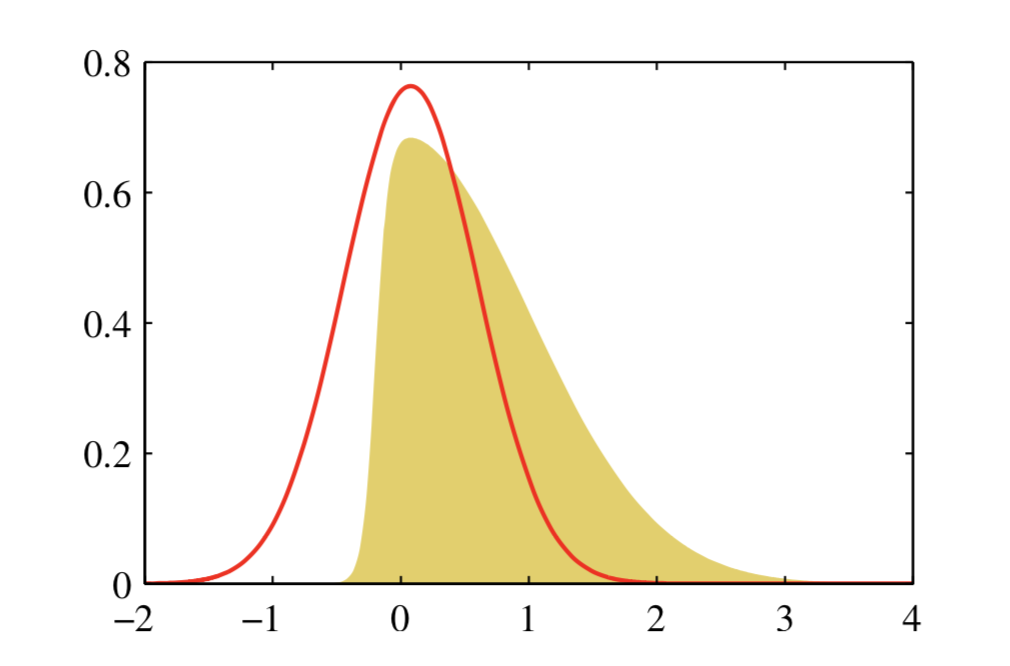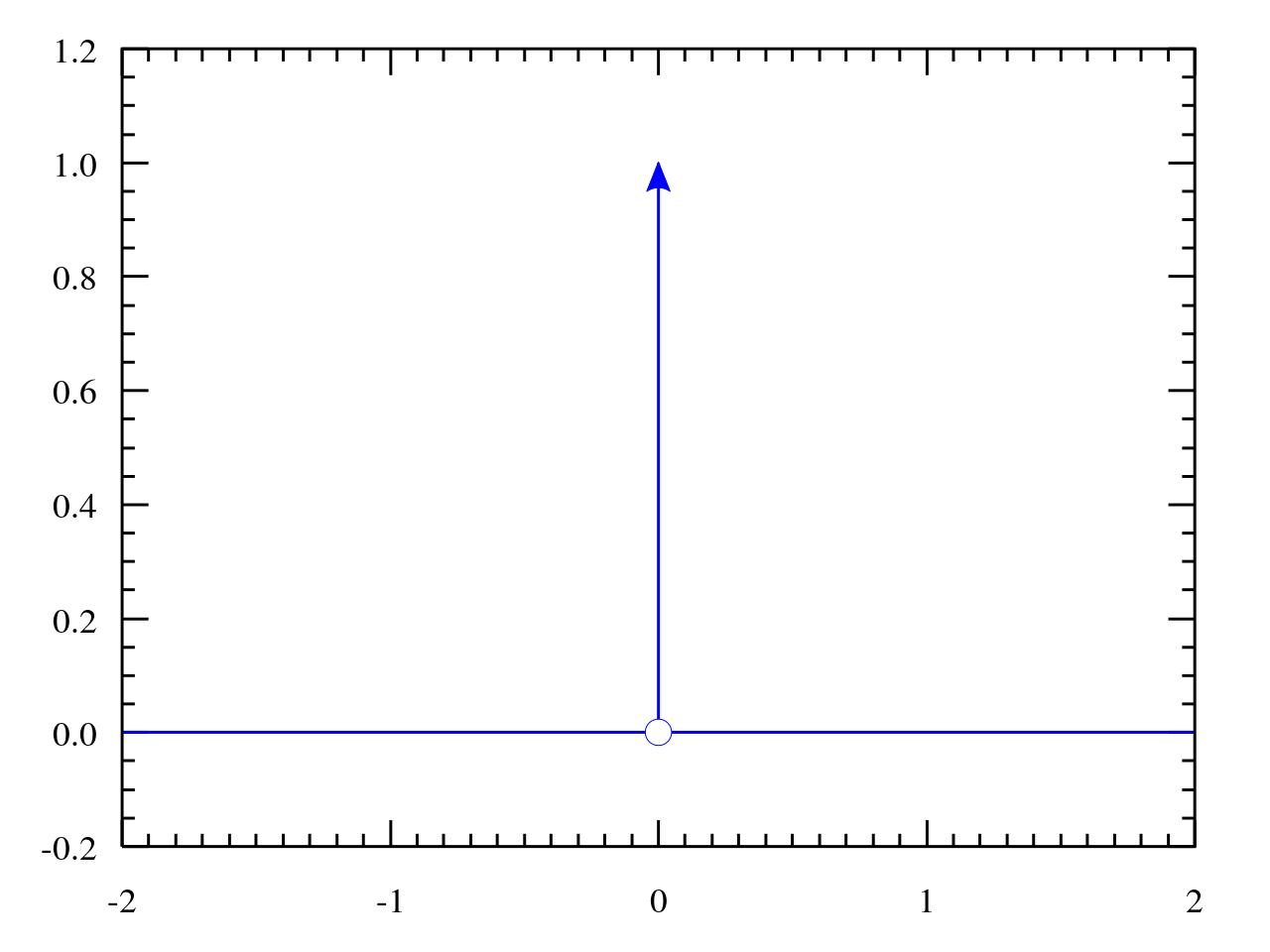## 拉普拉斯近似

$\mathbf{A}$需要是正定的。## 贝叶斯逻辑回归1. 因Dirac delta function可也看成一个高斯分布，$q(\mathbf{w})$也是高斯分布，高斯分布的边缘概率是高斯分布
2. 因Dirac delta function只在0处有值，其他地方均为0，$\delta(a-\mathbf{w^Tx})$可以看成对$\mathbf{w}$做了线性约束，$p(a)$可看成从联合概率密度$q(\mathbf{w})$中积掉了一部分，也是求边缘概率，所以也是高斯分布。（这一点是书上的解释，尚未理解透彻）